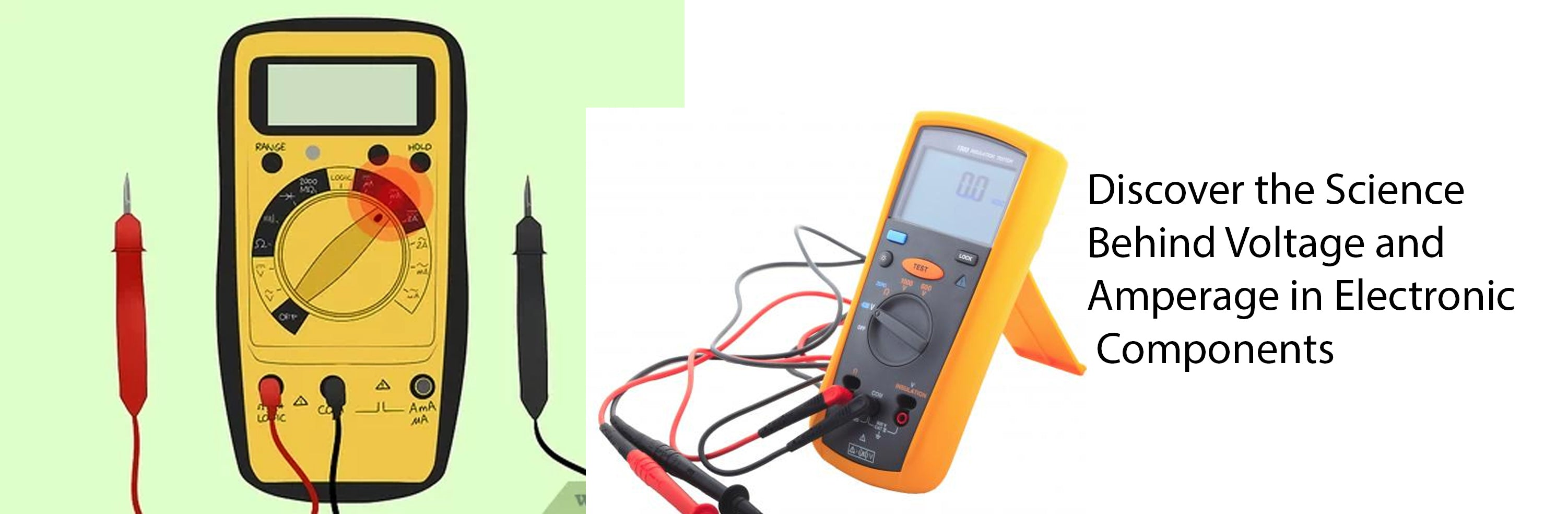## CATEGORIESDiscover the Science Behind Voltage and Amperage in Electronic Components

## What is Voltage and Amperage?

Before we dive into determining the voltage and amperage of an electronic component, let's understand what these terms mean. Voltage refers to the electric potential difference between two points, which determines the force that drives electric current. Amperage, on the other hand, measures the flow of electric current in a circuit. Both voltage and amperage are crucial in understanding the power requirements of electronic components.

## Checking the Component's Specifications

The easiest way to determine the voltage and amperage of an electronic component is by referring to its specifications. Most components, such as resistors, capacitors, and integrated circuits, come with datasheets that provide detailed information about their electrical characteristics. Look for the section that mentions voltage and amperage ratings.

## Using a Multimeter

If the component doesn't have readily available specifications or you want to verify the readings, you can use a multimeter. Set the multimeter to the appropriate voltage measurement range and connect the probes to the component's terminals. The multimeter will display the voltage across the component.

Similarly, to measure the amperage, you need to set the multimeter to the current measurement mode and connect it in series with the component. This way, the multimeter measures the current flowing through the component.

## Calculating Power Consumption

Once you have determined the voltage and amperage of the electronic component, you can calculate its power consumption. Power is measured in watts and is calculated by multiplying the voltage and amperage values. For example, if a component has a voltage of 12 volts and an amperage of 0.5 amps, the power consumption would be 6 watts (12V * 0.5A = 6W).

## Considerations and Precautions

When working with electronic components, it's important to consider a few factors. First, ensure that the power supply you are using matches the voltage requirements of the component. Using a higher voltage can damage the component, while a lower voltage may not provide sufficient power.

Additionally, be cautious when measuring amperage, especially in circuits with high currents. Using a multimeter with a low amperage rating can lead to inaccurate readings and potential damage to the multimeter or the component.

## Conclusion

Determining the voltage and amperage of an electronic component is crucial for understanding its power requirements. By referring to the component's specifications or using a multimeter, you can accurately measure these values. Remember to calculate the power consumption and consider the voltage and amperage ratings when working with electronic components. Following these steps will help you make informed decisions and ensure the proper functioning of your electronic projects.